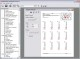Mathematics Worksheet Factory 3.1 A powerful, easy-to-use math worksheet generation program. SharewareLabMathX 1.0 LabMathX is a program for advanced mathematics, data display, and data archiving. SharewareEasy Calculat X 2.0.1 Easy Calculat is a calculator that can do simple calculations such and slightly more advanced mathematics like trigonometry. FreewareMath-Flight 2 2.3 Fly High while having fun learning basic mathematics with Math Flight! Shareware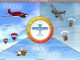Math Flight 2.3 Fly High while having fun learning basic mathematics with Math Flight! Shareware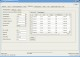ZinsMath 4.01 financial mathematics Shareware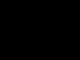Math Resource Studio 4.4.2 A powerful, easy-to-use math worksheet generation program. DemoMathDoggerX 0.0.1 MathDoggerX is a very very very simple Mathematics tutor. Freeware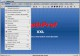MathProf 4.0 MathProf is an easy to use mathematics program within approximately 180 subrouti SharewareWorkSheet Maker 2.3.3 WorkSheet Maker is a teaching tool for mathematics. Freeware